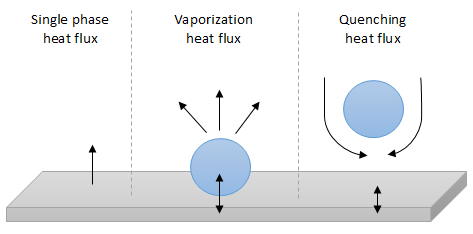# Nucleate boiling model

A ﬁrst model for nucleation mechanisms similar to the one developed by Frost and Dzakowik (1967)  is implemented in NEPTUNE_CFDThe single phase temperature proﬁle in the viscous sub-layer allows to obtain the relation between the wall superheat at boiling incipience ($T_{wall}-T_{sat}$) and the wall heat ﬂux ($Q_{wall}$), used as criteria for estimating the point at which nucleate boiling starts. For, $r_{Cmax}$ the maximum cavity radius available for activation on the wall, two criteria can be considered:

$T_{crit1}=T_{wall}-T_{sat}=(\frac{8 \sigma T_{sat } Q_{wall}}{\lambda_l \rho_{sat}H_{lat}})^{1/2}$   (18)

$T_{crit2}=\frac {Q_{wall}}{\lambda_l}r_{Cmax}+\frac{2\sigma T_{sat}}{h_{lg}\rho_{sat}}\frac{1}{r_{Cmax}}$   (19)

$r_{cl}=\frac{\lambda_l T_{crit1}}{2Q_{wall}}$   (20)

Starting the boiling process follows the subsequent pattern:

​if $r_{cl}\le r_{Cmax}$

$T_{wall}-T_{sat}=T_{crit1}$   (21)

Else, for $r_{cl}\ge r_{Cmax}$

$T_{wall}-T_{sat}=T_{crit2}$   (22)

Three-flux model

In a ﬁrst simpliﬁed approach, the boiling heat ﬂux is calculated following the analysis of Kurul and Podowski (1990) , ​, where $Q$ it is split into three terms, a single phase ﬂow convective heat ﬂux $Q_c$ at the fraction of the wall area unaffected by the presence of bubbles, a quenching heat ﬂux $Q_q$ where bubble departures periodically bring cold water in contact with the wall and finally, a vaporization heat ﬂux $Q_e$ needed to form the vapor phase (figure 4).

$Q=Q_c+Q_q+Q_e$   (23)Figure 4: Analysis of Kurul and Podowski (1990) for the boiling heat flux

The wall surface unit is split into two parts: an area inﬂuenced by bubble departure, neglecting the overlapping areas of inﬂuence between adjacent bubbles, defined as $A_q=min(1,\frac{\pi D_m^2n}{4})$ and a single phase area $A_c$ with the relation $A_c+A_q=1$$D_m$ represents the departure bubble diameter [m] and $n$, the active site density [number/m2].

The convective heat ﬂux is calculated with the classical law of single phase ﬂow heat transfer at the wall

$Q_c=A_c h_{log}(T_{wall}-T_{\delta})$   (24)

where $\delta$ refers to a point in the ﬂuid at a dimensionless distance from the wall and $h_{log}$ represents the heat transfer coefficient within the thermal boundary layer.

The quenching heat ﬂux is modelled as the mean value of a transient conductive heat ﬂux supplied to a medium at external temperature $T_{\delta}$, during the period $t_Q$ between the departure of a bubble and the beginning of growth of the following one

$Q_q=A_Qt_Qf \frac{2\lambda_l(T_{wall}-T_{\delta})}{(\pi\alpha_lt_Q)^{1/2}}$   (25)

$\alpha_l$ and $f$ represent the liquid thermal diffusivity and the bubble departure frequency, respectively.

Finally, the vaporisation heat is expressed as follows

$Q_e=fV_b\rho h_{lg}n$   (26)

Proportional to the active site density $n$ and the bubble's volume $V_b=\frac{\pi D_m^3}{6}$

In order to run the simulation, closures laws as the mean bubble equivalent diameter, the mean bubble departure frequency and the active site spatial density, should be provided. The usual closures laws used in NEPTUNE_CFD are the following ones:

• Mean bubble equivalent diameter (Unal -1976 )

$R_d=\frac{1,2.10^5P^{0.709}a}{\sqrt{b\Phi}}$   (27)

Where,

• $a=\frac{T_{wall}- T_{sat}}{2\rho_gh_{lg}} \sqrt{\frac {\lambda_{wall}\rho_{wall}Cp_p}{\pi}}$,   (28)
• $b=\frac{T_{sat}-T_l}{2(1-\frac{\rho_g}{\rho_l})}$   (29)
• $\Phi=max(1,(\frac{U_l}{0.61})^{0.47})$   (30)

• Frequency (Ceumern & Lindenstjerna -1977 ):

$f=\sqrt{\frac{2g(\rho_l-\rho_v)}{3\rho_lR_d}}$   (31)

$t_Q = 1/f$   (32)

• Active site spatial density (Lemmert et Chwala -1977 )

$n=(210(T_{wall}-T_{sat}))^{1.8}$   (33)

Four-Flux Model

The basic wall heat flux partitioning model assumes that the amount of liquid on the wall is sufficient to remove heat from the wall to be used for evaporation. Thus, superheating of the vapour that occurs at high void fractions is not modelled. Under these assumptions, the basic heat flux partitioning model cannot be used, and a generalised model is considered. In this model, the total heat flow is considered to be composed of four different contributions, however, the criteria for onset of nucleated boiling remains unchanged.

$Q=Q_c+Q_{c2}+Q_q+Q_e$   (34)

The main differences between the three-flux and the four-flux models are listed below:

• The monophasic heat flux in the liquid phase $Q_c$ is not weighted by $A_c$ (the area of the wall unaffected by the bubbles) but by the volume fraction of the liquid $\alpha_l$.
• A new term $Q_{c2}$ is considered, which represents the convective heat transfer between the wall and the vapour phase. It is weighted by the vapour volume fraction $\alpha_v$.

$Q_{c2}=h_{wfv}(T_{wall}-T_{v})$   (35)

Where $h_{​wfv}$ is the wall heat transfer coefficient calculated from the wall temperature function for the vapour phase and $T_v$ is the vapour temperature at the centre of the wall adjacent cell.

• In the previous model, $Q_q$ was calculated by considering the liquid as a semi-infinite medium. In this new model, it is weighted by the volume fraction of liquid into the first mesh, since it would not exist if there is no new cold liquid in contact the wall.
• The density of active cavities is modified to take into account the different boiling regimes. This equation has been obtained by modifying the equation given by Kurul & Podowski for nucleate boiling to: $n=min((210(T_{wall}-T_{sat})^{1.8},n_{max})$ where $n_{max}$ corresponds to a surface completely covered with bubbles.

This model seems to be more robust than the tree-flux model, and thus to provide better results when high heat flux are considered. The extension of the wall-heat-flux-partitioning model was used also to take into account the critical heat flux condition.